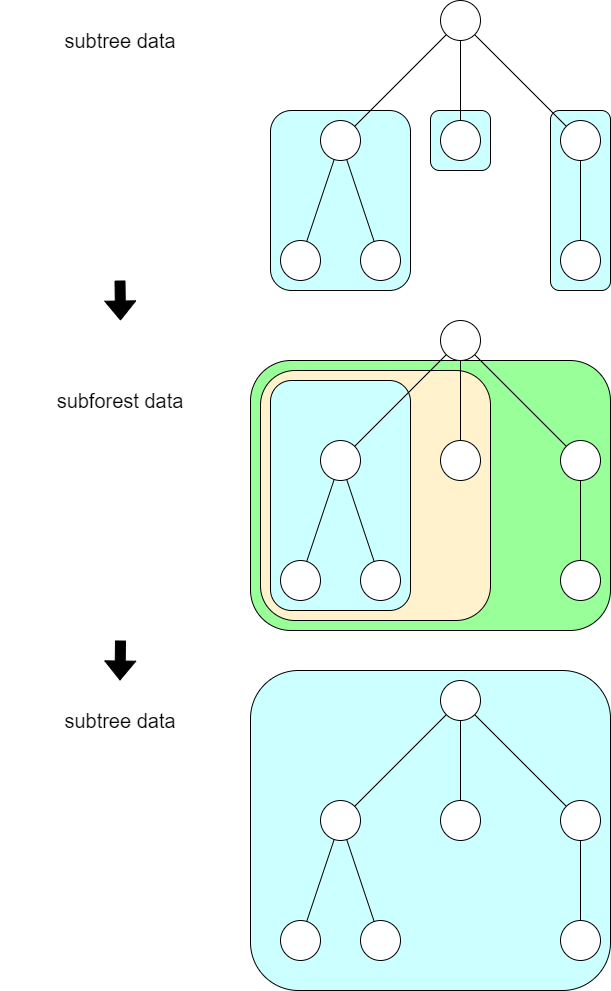## D - Zigzag Tree 解説 by evima

Let us choose the root arbitrarily so that the graph is rooted.

Assigning a permutation to the vertices can be rephrased into setting a total order on the vertices. The condition in question holds if and only if one of the following holds for any vertex $$v$$ (the inequality is regarding the total order):

• $$v > w$$ for any $$w$$ adjacent to $$v$$,
• $$v < w$$ for any $$w$$ adjacent to $$v$$.

Let us say a $$v$$ with the former property big, and a $$v$$ with the latter property small.

### ◆ Tree DP

For a rooted subtree with $$n$$ vertices rooted at $$v$$, let us call the following value $$\mathrm{dp}_v[i]$$.

• The number of ways to set a total order on the vertices in the rooted tree $$v$$ such that the root $$v$$ is small and the value assigned to the root is the $$i$$-th smallest value.

We can solve the problem by a tree DP that computes this from bottom to top. In this DP, we first need to merge the information from subtrees to compute the information for subforests in order.For a forest with $$n$$ vertices, we compute the following for each $$i$$.

• The number of ways to set a total order on the vertices in the forest such that all roots are small and the maximum value assigned to the root is the $$i$$-th smallest value.

### ◆ Merging the DP table

We can see that computing the following is the key to the problem.

• We have a set $$A$$ with $$n$$ elements and a set $$B$$ with $$m$$ elements.
• $$\mathrm{dp}_A[i]$$ is given, which is the number of total orders on $$A$$ where the root is the $$i$$-th smallest and a certain requirement is satisfied. $$\mathrm{dp}_B[i]$$ is given similarly.
• Compute $$\mathrm{dp}[i]$$, the number of total orders on $$A\amalg B$$ where the restrictions on $$A$$ and $$B$$ satisfy the requirement and the larger of the roots of $$A$$ and $$B$$ is the $$i$$-th smallest.

We will divide the computation into two, based on which of the roots is larger.

Let us fix the order $$a_1 < a_2 < \cdots < a_n$$ on $$A$$ and the order $$b_1 < b_2 < \cdots < b_m$$ on $$B$$. When the root of $$A$$ is $$A_i$$, the number of ways to extend the orders so that $$a_i$$ is the $$(A\amalg B)$$-th smallest in $$A\amalg B$$ can be computed as the product of the following:

• setting the order between $$a_1,\ldots,a_{i-1}$$, $$b_1,\ldots,b_j$$: $$\binom{i-1+j}{i-1}$$ ways
• setting the order between $$a_{i+1},\ldots,a_n$$, $$b_{j+1},\ldots,b_m$$: $$\binom{n-i+m-j}{n-i}$$ ways

Additionally, $$a_i$$ is larger than the root of $$B$$ when the root of $$B$$ is one of $$b_1,\ldots,b_j$$. Thus, to $$\mathrm{dp}[i+j]$$, we will add $$\binom{i-1+j}{i-1}\binom{n-i+m-j}{n-i}\mathrm{dp}_A[i] (\mathrm{dp}_B+\cdots+\mathrm{dp}_B[j])$$. After pre-calculation of prefix sums, this can be done in $$O(nm)$$ time in total.

### ◆ Complexity Analysis

In a tree DP, if merging the information of subforests of sizes $$n$$ and $$m$$ takes $$O(nm)$$ time, the information of the whole rooted tree with $$N$$ vertices can be computed in $$O(N^2)$$ time. The analysis of this complexity can be found, for example, on:

This analysis or tree DPs that involve it is often called “squared tree DP” in the Japanese kyo-pro community.